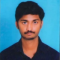## If $x^{2}+\frac{1}{x^{2}}=18$, find the values of $x+\frac{1}{x}$ and $x-\frac{1}{x}$.Akhileshwar Nani

Updated on 01-Apr-2023 11:59:56

35 Views

Given:$x^2 + \frac{1}{x^2} = 18$To do:We have to find the values of $x + \frac{1}{x}$ and $x - \frac{1}{x}$.Solution:The given expression is $x^2 + \frac{1}{x^2} = 18$. Here, we have to find the values of $x + \frac{1}{x}$ and $x - \frac{1}{x}$. So, by using the identities $(a+b)^2=a^2+2ab+b^2$...................(i) and $(a-b)^2=a^2-2ab+b^2$.............(ii), ... Read More

## Find the value of $x$, if:(i) $4x = (52)^2 – (48)^2$(ii) $14x = (47)^2 – (33)^2$(iii) $5x = (50)^2 – (40)^2$Akhileshwar Nani

Updated on 01-Apr-2023 11:51:59

127 Views

Given:(i) $4x = (52)^2 – (48)^2$  (ii) $14x = (47)^2 – (33)^2$  (iii) $5x = (50)^2 – (40)^2$To do:We have to find the value of $x$ in each case.Solution:Here, we have to find the value of $x$ in each expression. The given expressions are the difference of two squares. So, ... Read More

## Simplify the following using the formula: $(a - b) (a + b) = a^2 - b^2$:(i) $(82)^2 – (18)^2$(ii) $(467)^2 – (33)^2$(iii) $(79)^2 – (69)^2$(iv) $197 \times 203$(v) $113 \times 87$(vi) $95 \times 105$(vii) $1.8 \times 2.2$(viii) $9.8 \times 10.2$Akhileshwar Nani

Updated on 01-Apr-2023 11:50:10

320 Views